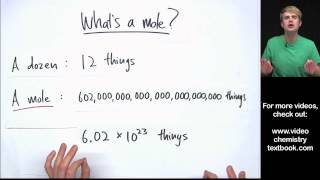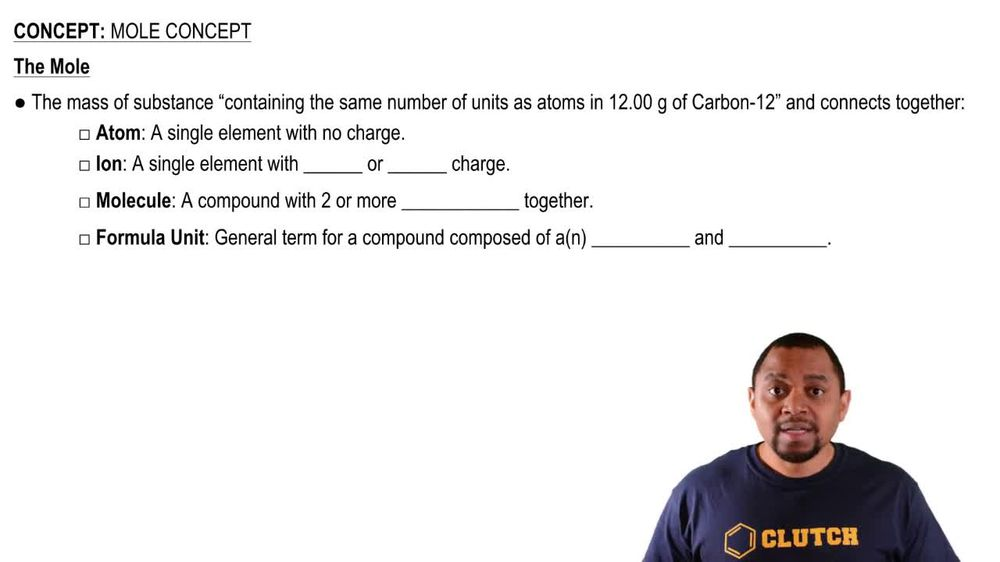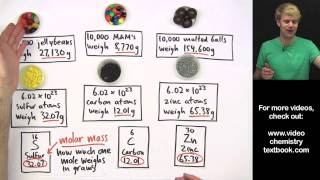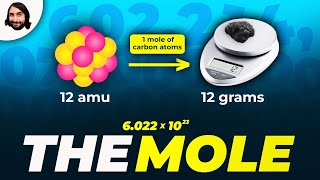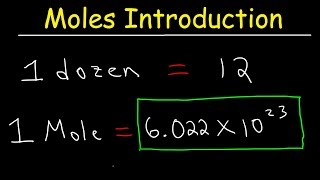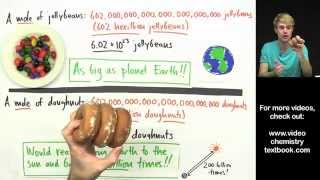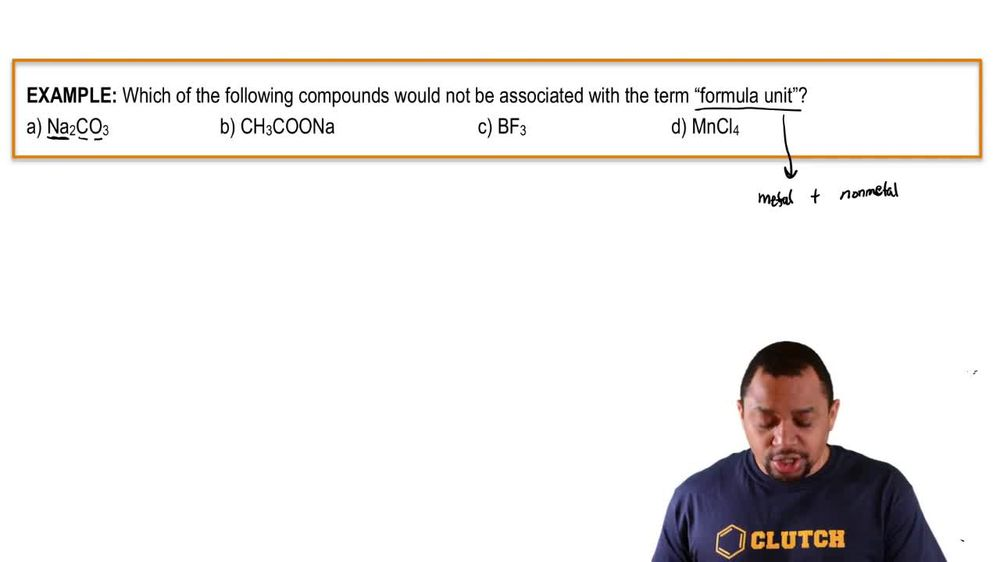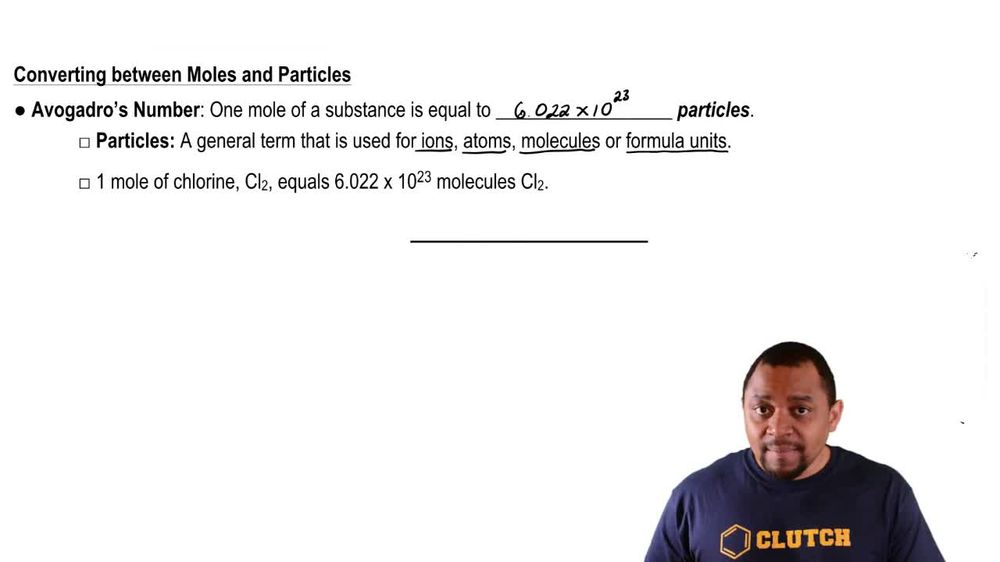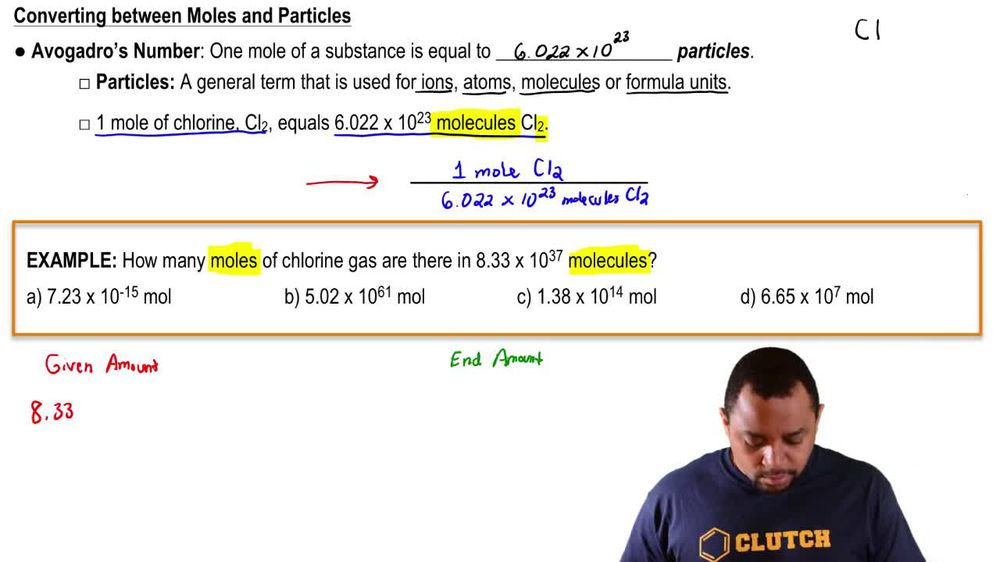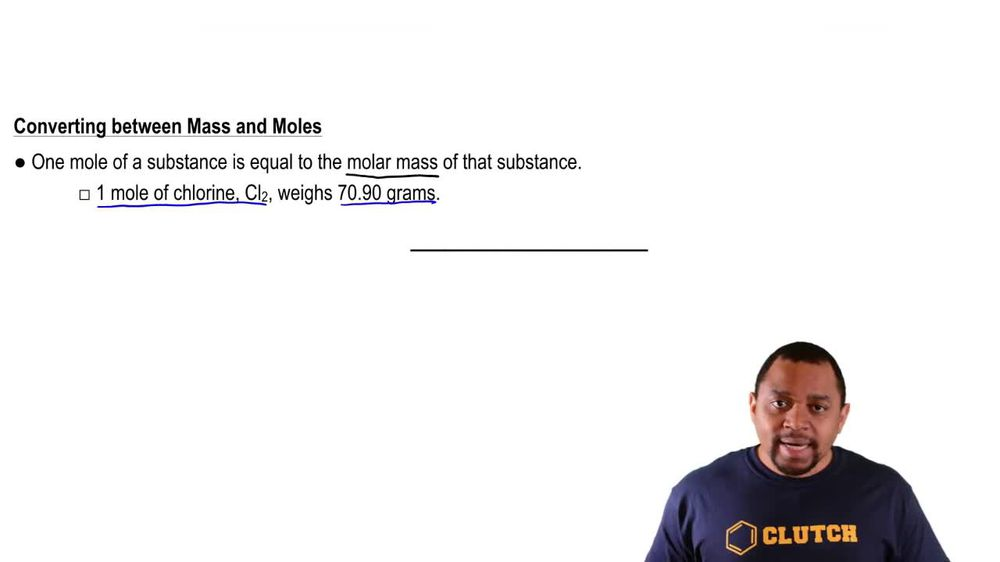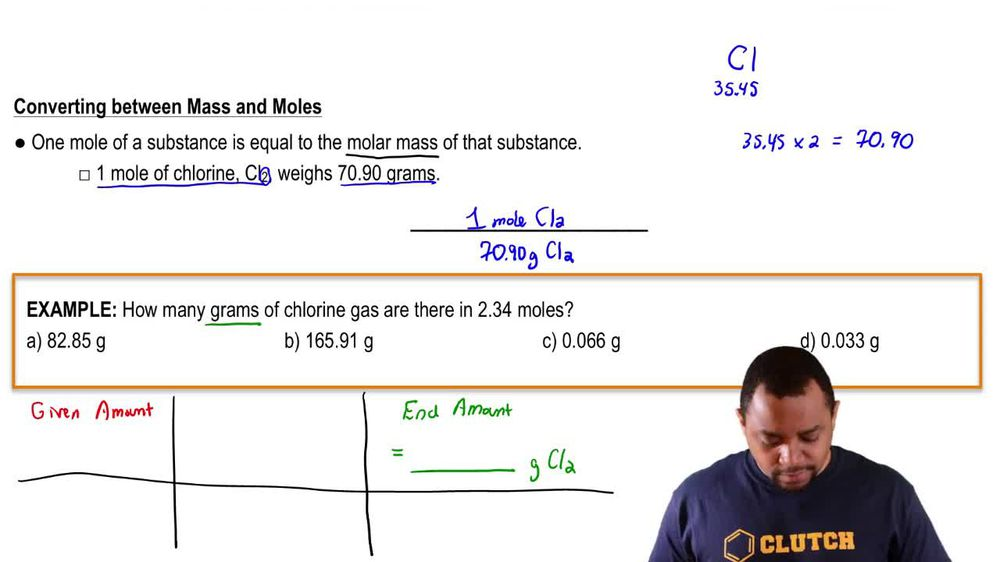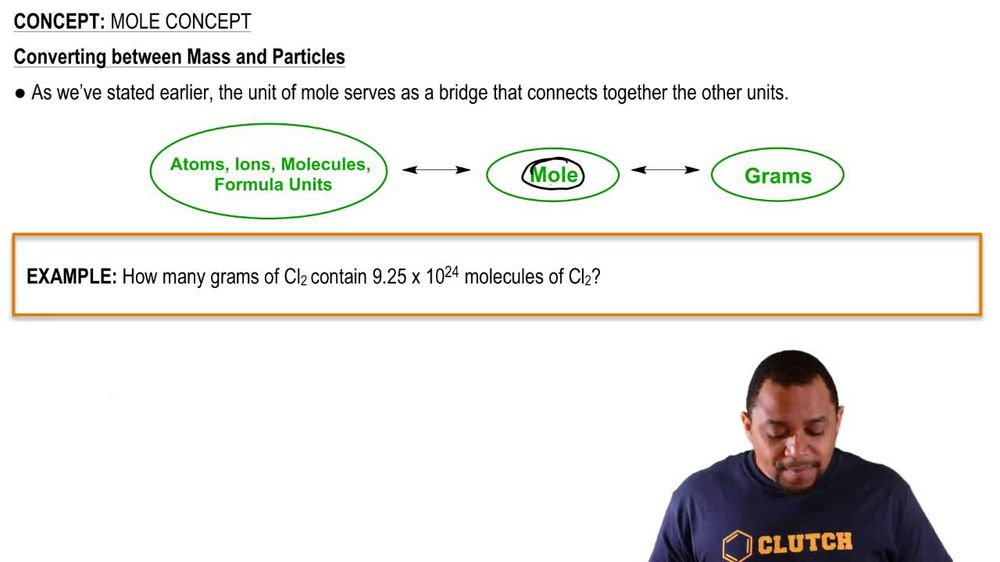Start typing, then use the up and down arrows to select an option from the list.
1. 2. Atoms & Elements2. Mole Concept# Mole Concept Example 4

by Jules Bruno
106 views
0
As we stated earlier, the unit of moles can act as a bridge that connects together the other units. So here we can see that moles rise right in the middle between grams and all the terms that we collectively call particles. Now, if we take a look here at this example question it says how many grams of seal to contain 9.25 times 10 to the 24 molecules off all too. All right, so we're going to start out with are given amount, which is 9.25 times 10 to the molecules off CL too. And we have to get to our end amount over here. Now, remember, we're going to say, in order to get there, we're gonna have to utilize some conversion factors and our end amount that we want to get to is grams off. CL too. So if we take a look here, we need to get rid off molecules first. So our first conversion factor, we're going to say that we do 6.22 times 10 to the molecules of C l two. I remember that connects us to moles, so for every one mole of C l two. Then we're going to realize that molecules air out. Now we have moles. We're not there yet, though, because we don't want moles at the end as our answer, we want grams. So our second conversion factor, which we saw earlier, would be that one mole of CL to here on the bottom is 70.90 g of C l two here on the top. Here are moles would cancel out, and we have our answer Ingram's. Now, when you do the math, make sure you put these in parentheses. Otherwise, your calculator may give you the incorrect answer. So we do. 9.25 times 10 to 24 times 70.90 divided by avocados Number here. Now, if you did this correctly, what you get initially is 1089.48 g off. CL too. But realize at this point we have three sig figs in our original value given to us. So we need this answer also to be in three sig figs. So we write that in scientific notation, asked one point 100 actually, we could do 1.9 times 10 to the g of C. L two. So here, writing in scientific notation gives us our three significant figures, which we had in the original question. 9.25 times 10 to the 24 given to us has three sig figs. So are answered. Must have three sig figs.